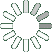Puzzle Command
Daily Puzzle
Number Logic #26
April 26, 2015Find a 6 digit number where:

The 1st digit is any number. The 2nd digit is equal to 8. The 3rd digit is the number of 7s in the number and equal to 1. The 4th digit is the number of 7s in the number. The 5th digit is the sum of the 1st and 4th digit. The 6th digit is an odd number.

Puzzle Notes
6 digit numbers range from 100,000 to 999,999.
Enter in your solution below and click submit.